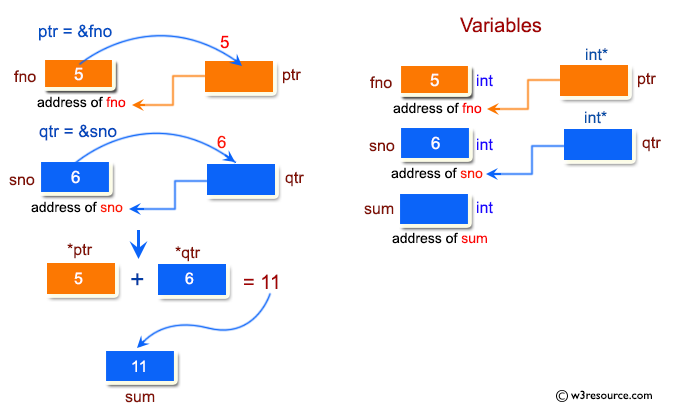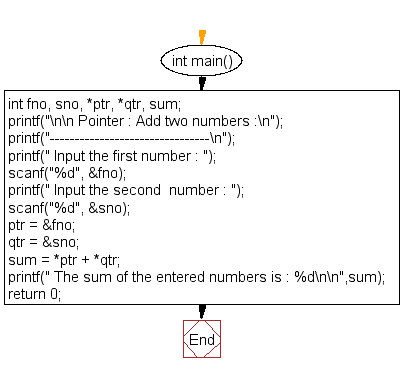﻿ C Program: Add two numbers - w3resource# C Exercises: Add two numbers

## C Pointer : Exercise-4 with Solution

Write a program in C to add two numbers using pointers.

Pictorial Presentation:Sample Solution:

C Code:

``````#include <stdio.h>
int main()
{
int fno, sno, *ptr, *qtr, sum;

printf("\n\n Pointer : Add two numbers :\n");
printf("--------------------------------\n");

printf(" Input the first number : ");
scanf("%d", &fno);
printf(" Input the second  number : ");
scanf("%d", &sno);

ptr = &fno;
qtr = &sno;

sum = *ptr + *qtr;

printf(" The sum of the entered numbers is : %d\n\n",sum);

return 0;
}
```
```

Sample Output:

``` Pointer : Add two numbers :
--------------------------------
Input the first number : 5
Input the second  number : 6
The sum of the entered numbers is : 11
```

Flowchart:C Programming Code Editor:

Have another way to solve this solution? Contribute your code (and comments) through Disqus.

What is the difficulty level of this exercise?

Test your Programming skills with w3resource's quiz.

﻿

## C Programming: Tips of the Day

How to format strings using printf() to get equal length in the output?

You can specify a width on string fields, e.g.

`printf("%-20s", "initialization..."); `

And then whatever's printed with that field will be blank-padded to the width you indicate.

The - left-justifies your text in that field.

Ref : https://bit.ly/34DMOc3